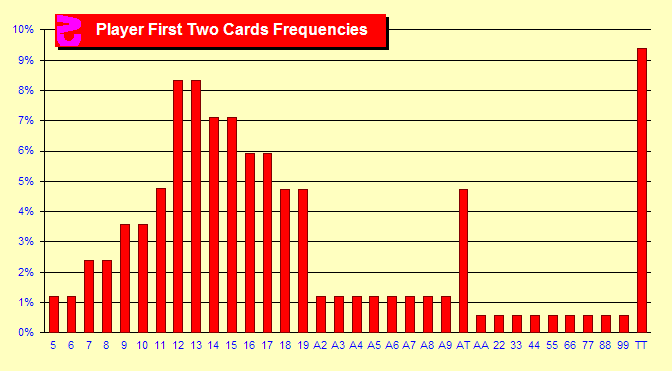# Chapter 1. Basic Strategy

Every complex concept is built upon simple concepts. If we do not have a solid understanding of the simple underpinnings of a subject, the complex concepts are more difficult to absorb. So, let us start out with some simple charts.

"I'll know my song well before I start singing" — Bob Dylan, 1962

###1.1 What hands do we get?

There exist 34 different hands that you can draw at the start of a Blackjack round. You can have a hard count of 5 through 19, a soft count of A,2 through A,T, or a pair of Aces through Tens. (Note: A hard count of 2 would always be a pair of Aces and a hard count of 4 would always be a pair of Deuces. There is no hard count of 3 as an Ace, Deuce is a soft count. A hard count of 20 would always be a pair of Tens.)

As you can see from the chart at the right, the percentage of the time that you find yourself with a particular hand varies dramatically. This chart is for six decks. Pairs are not common as less than 1% of the time you will receive a specific pair of Aces through nines. The exception is a pair of Tens. Happily, this is the most common hand since tens include Jacks, Queens and Kings. Soft counts are also not that common at a bit more than 1% chance of any specific soft count. Again happily, A,T (Blackjack) is more common at about 4.75% due to the large number of Tens in a deck. The bad news is that stiffs (hands that can be busted with one draw) are very common: 12 & 13 at over 8% each and 14, 15 and 16 at 7% or 6% each. This is because there are many combinations of cards that add to totals of 12 through 16.

###What about single-deck Blackjack?

Single-deck and six-deck games do not have the same frequencies of hands. The second chart shows the differences in frequencies between SD and 6D. Wherever you see a bar pointing up, that hand is more common in single deck. If the bar points down, the hand is more common in shoes. For example, with single deck you will notice that there are fewer pairs than with six decks. This is simple combinatorial probability. In single deck, if you have a six there are only three sixes left that you can draw as a pair or 3/51 (5.9% probability). With six decks there are 23 sixes left or 23/311 (7.4% probability). Blackjacks are more common in single deck. 4.827% vs. 4.749%. That doesn't sound like much difference, but considering the importance of Blackjacks it is a major factor in the advantage difference in number of decks. Unfortunately, single deck also sees more stiffs (12-16).

### Sim details

• Six decks, S17, DAS, LS, Heads-Up, Basic Strategy, 75% Penetration
• Single-deck, H17, nDAS, Heads-Up, Basic Strategy, Six Rounds
• Ten billion rounds each